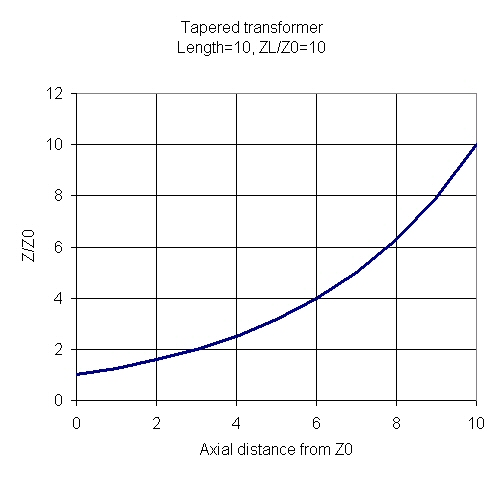# Tapered Transformers

New for September 2021: here's an example of an equal-ripple multi-section transformer using a spreadsheet you can download from our site.

This page will discuss exponential and Klopfenstein (and possibly other) tapered transformers. Transformers are used to match one impedance to another (from Z1 to Z2). In most cases, the impedances are purely real (not inductive or capacitive). By tapering a transmission line, a very broadband impedance match (low VSWR) can be realized over a wide bandwidth, the longer the taper, the wider the frequency band.

### Exponential transformer

In the exponential taper, the natural logarithm of the line's characteristic impedance varies linearly from Z1 to Z2:

ln(Z/Z0)=(z/L)xln(ZL/Z0)

Where z is the distance from the Z0 side of a transformer that has length L.

Perhaps a better way to look at this is that the line impedance increases exponentially according to:

(Z/Z0)=(ZL/Z0)^(z/L)

Here's a plot of the line impedance of a tapered transformer of length 10 (units are arbitrary) that is being used to match ZL/Z0=10.We'll add some more math here so you can see what the impedance looks like over frequency.

### Klopfenstein transformer

This topic was contributed by someone at Endwave who prefers not to have his name attached to it. Thanks, Mark! We plan to expand on it in the future, after we look up the 51 year old article.

In the January 1956 Proceedings of the IRE, R. W. Klopfenstein presented equations which can be used to design transmission line tapers. "...the Dolph-Tchebycheff taper is optimum in the sense that it has minimum reflection coefficient magnitude in the passband for a specified length of taper". One drawback of the Klopenstein taper is that an impedance discontinuity or step occurs at the ends of the taper.

A Mathcad routine for synthesizing the dimensions can be found at: